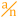# Modulo

 Block Group: Math Operations Icon:The Modulo block returns the quotient and remainder from division.

For information on using dataflow blocks, see Dataflow.

For accepted and excluded arguments of Math Operations blocks, see Math Operations.

## Input/Output Properties

The following properties of the Modulo block can take input and give output.

• input (number)
• modulo (number)

input specifies the dividend.

modulo specifies the divisor.

## Output Properties

The following properties of the Modulo block can give output but cannot take input.

• quotient (number)
• remainder (number)

quotient returns the integer result of input divided by modulo.

remainder returns the remainder. The remainder property always has the same sign as the input property.

## Examples

The following image shows five examples of the Modulo block.